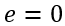# Orbital Speed for All Conic Sections

### Specific Energy for Two-Body Orbit

Specific energy is provided, without proof, in Eq. (1) for where specific energy is a constant for any conic section. Derivation will be provided in future articles.(1)

Specific energy is further reduced for all conic sections in Eq. (2) as a function of the gravitational parameter for the central body and the semimajor axis of the orbit.(2)

### Orbital Speed for All Conic Sections

#### Elliptical and Circular

Using the specific energy equations (1) and (2), we can calculate the speed at some distance, r, from the focus as shown in Eq. (1).(3)

There is no variation in the distance from the focus in a circular orbit,. Reducing Eq. (3) to Eq. (4), we only need to know the circular orbit radius and gravitational parameter of the central body. The circular orbit radius is equal to the semimajor axis.(4)

#### Parabolic

A parabolic orbit represents the line between closed and open conic orbits. A probe given sufficient escape speed will travel on a parabolic escape trajectory from the central body. As the probe’s distance from the central body increases the speed necessary to escape decreases to zero. We determine the escape speed by comparing the specific energy of two points along this theoretical escape trajectory.(5)

#### Hyperbolic

Using the same trick as a parabolic orbit for the hyperbolic orbit, we must account for the excess hyperbolic speed at some infinite distance.(6)